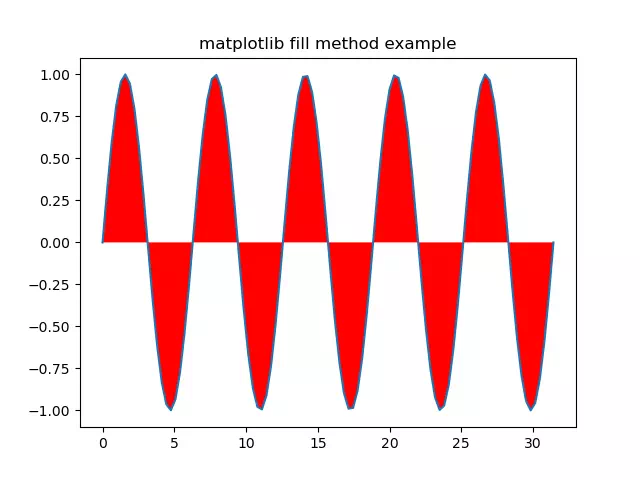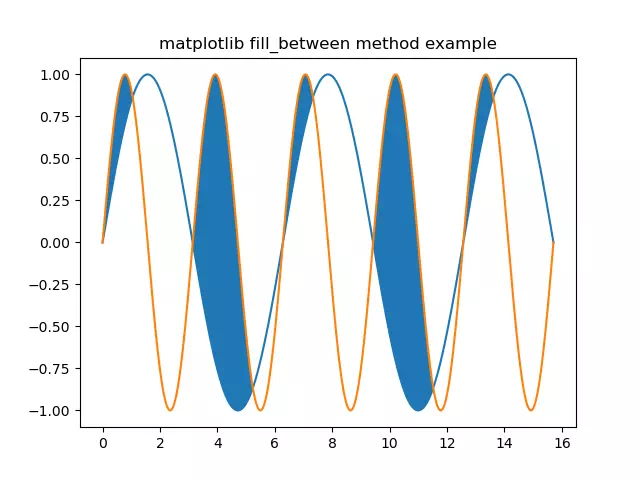# Matplotlib fill & fill_between Example

This article will tell you how to use the Matplotlib fill() method to fill the Matplotlib curve colors and how to use the fill_between() method to fill the colors between 2 Matplotlib curves.

### 1. Matplotlib fill() Method Example.

1. The fill(x, y, color) method needs at least 3 parameters to fill the curve color.
2. x: the x-axis values array.
3. y: the y-axis values array.
4. color: the curve-filled color string such as ‘r’ means red color.
5. You can refer to the matplotlib.pyplot.fill to get the complete parameters list.
6. Below is the example source code.
```import numpy as np
import matplotlib.pyplot as plt

def fill_example():

# set the curve title.
plt.title('matplotlib fill method example')

# create an array, starts with 0, ends with 10*np.pi, create 1000 elements in the array.
x_array = np.linspace(0, 10*np.pi, 100)

print('x_array: ', x_array)

print('len(x_array): ', len(x_array))

# calculate the  y array values.
y_array = np.sin(x_array)
print('y_array: ', y_array)

# plot the  curve.
plt.plot(x_array, y_array)

# fill the curve with red color.
plt.fill(x_array, y_array, 'r')

plt.show()

if __name__ == '__main__':

fill_example()```
7. Below is the above source code generated figure.### 2. Matplotlib fill_between() Method Example.

1. The fill_between(x, y1, y2, where, interpolate, step) method needs at least 6 parameters to fill color between 2 Matplotlib curves.
2. x: the x-axis values array.
3. y1: the first curve’s y-axis values array.
4. y2: the second curve’s y-axis values array.
5. where: the filled area that matches this condition.
6. You can get the complete parameter introduction from the matplotlib.pyplot.fill_between official document.
7. Below is the example source code.
```import numpy as np
import matplotlib.pyplot as plt

def fill_between_example():
# set the curve title.
plt.title('matplotlib fill_between method example')

# create x axis value array, starts from 0, ends with 5*np.pi, total generate 1000 array elements.
x_array = np.linspace(0,5*np.pi,1000)

# calculate the first y axis value array.
y_array1 = np.sin(x_array)

# calculate the second y axis value array.
y_array2 = np.sin(2 * x_array)

# plot the first curve.
plt.plot(x_array, y_array1)

# plot the second curve.
plt.plot(x_array, y_array2)

# fill the color between the area where y_array1's value less than y_array2's value.
plt.fill_between(x_array, y_array1, y_array2, where=y_array1<y_array2, interpolate=True)

plt.show()

if __name__ == '__main__':

fill_between_example()```
8. When you run the above example source code, it will show the below figure.This site uses Akismet to reduce spam. Learn how your comment data is processed.